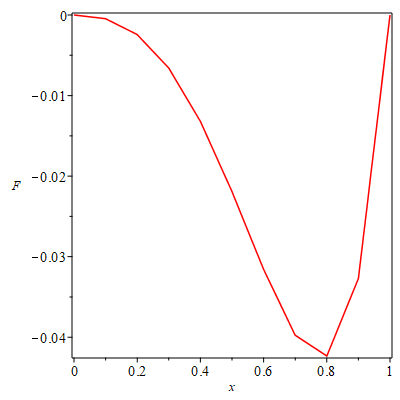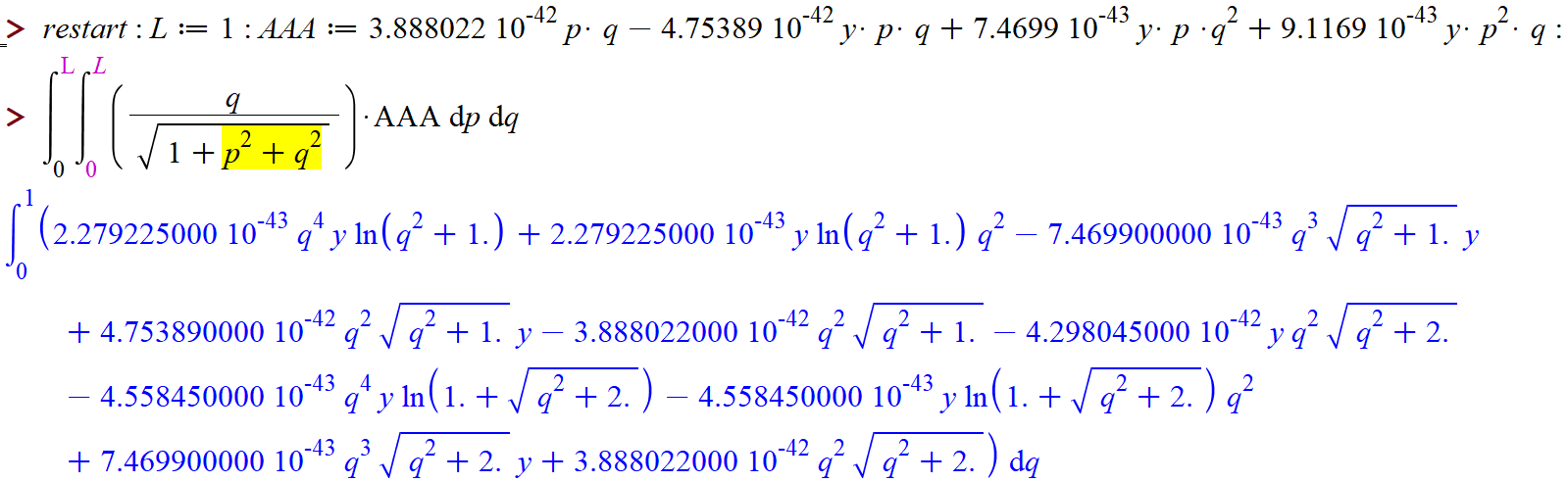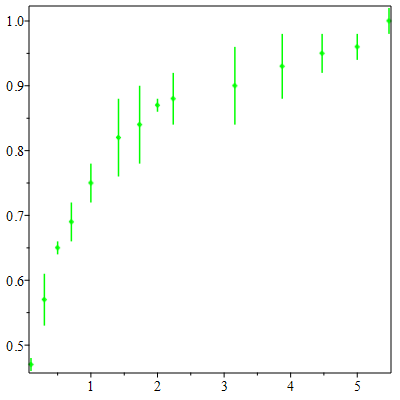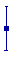## why .mla file remain locked open when using it?...

I noticed the following. I have 2 worksheets. A.mw and B.mw.  From A.mw, it does something as follows

```libname := currentdir(), libname
foo();```

Where foo() is entry inside my say TMP.mla in currentdir(). This works fine with no problems and foo() executes and complete OK.

Now I make changes to the code foo.mpl, and need to update TMP.mla. currently I use LibraryTools. But i'd like to delete TMP.mla each time and create new one so I am sure there is no problem. So from B.mw I run this code

```libname:= currentdir(),libname;
FileTools:-Remove("TMP.mla");
LibraryTools:-Create("TMP.mla");
LibraryTools:-Save('foo',"TMP.mla");```

This all works well, except it fails at the Remove line, since "TMP.mla" could not be removed, since it was open before when running the A workseet, and it seems to be still open and Maple could not delete the file.

So each time this happens, I close all worksheets, close Maple, open Maple again, and now I can run the above code OK to update the mla.

Is there a better way to do all of this?

I worry if I do not delete the mla each time, I could end up with old code there I do not want, if I rename things in my code. That is why I like to start with fresh mla file each time.  But I always have to restart Maple now to update the mla.

## International Mathematics Competition for University...

by: Maple

This year, the International Mathematics Competition for University Students  (IMC) took place online (due to Coronavirus), https://www.imc-math.org.uk/?year=2020

One of the sponsors was Maplesoft.

Here is a Maple solution for one of the most difficult problems.

Problem 4, Day 1.

A polynomialwith real coeffcients satisfies the equation, for all real.

Prove thatfor.

A Maple solution.

Obviously, the degree of the polynomial must be 101.

We shall find effectively p(x).

 > restart;
 > n:=100;(1)
 > p:= x -> add(a[k]*x^k, k=0..n+1):
 > collect(expand( p(x+1) - p(x) - x^n ), x):
 > S:=solve([coeffs(%,x)]):
 > f:=unapply(expand(eval(p(1-x)-p(x), S)), x);(2)
 > plot(f, 0..1); # Visual check: f(x)>0 for 0> f(0), f(1/4), f(1/2);(3)
 > sturm(f(x), x, 0, 1/2);(4)

So, the polynomial f has a unique zero in the interval (0, 1/2]. Since f(1/2) = 0  and f(1/4) > 0, it results that  f > 0 in the interval  (0, 1/2). Q.E.D.

## how to get fine cure in this figure?...

I need to get fine curved figure.

please suggest command.   Please also tell 6 differnt markers like symbol = asterisk.## Retrieve elements of lists of a list ignoring []...

Hello

Since my solutions are neither efficient nor concise, I wonder if someone could help me with the following problem. Suppose

`L:=[[[],[],3,2,1,4],[1,4,[],5,8,[]],[5,6,7,8,[],1]]:`

(Just a short and simplified version - L is a huge list). I need the elements to be rearranged as follows

`newL:=[[1,5],[4,6],[3,7],[2,5,8],[1,8],[4,1]]:`

that is, the first sublist of newL contains the first elements of all sublists of L without [], the second sublist of newL contains the second elements of all sublists of L without [] and so on.

How can that be achieved without seq and remove?

Many thanks

Ed

PS. My solution

`newL:=[seq(remove(y->y=[],map(x->x[i],L)),i=1..nops(L))]`

The size of the sublists does not change.

## Circumscribed ellipse

by: Maple 17

One way to find the equation of an ellipse circumscribed around a triangle. In this case, we solve a linear system of equations, which is obtained after fixing the values of two variables ( t1 and t2). These are five equations: three equations of the second-order curve at three vertices of the triangle and two equations of a linear combination of the coordinates of the gradient of the curve equation.
The solving of system takes place in the ELS procedure. When solving, hyperboles appear, so the program has a filter. The filter passes the equations of ellipses based on by checking the values of the invariants of the second-order curves.
FOR_ELL_ТR_OUT_PROCE_F.mw  ( Fixed comments in the text  01, 08, 2020)## Calculus of variations for a deformable surface. h...

Hello everyone.

I am not really sure how to solve the following problem:

Given a parametriaztion of a surface as an interpolation in terms of given nodes as:
X(ξ,η)= Σ Νi(ξ,η) Xi, it is possible to calculate tangent and normal vectors as:
α1(ξ,η)= Σ Νi,ξ(ξ,η) Xi     α2(ξ,η)= Σ Νi,η(ξ,η) Xi  and a3(ξ,η)= cross(a1,a2) and their derivatives
α1,ξ α1,η α2,ξ α2,η etc.
For a variation of Xi i.e.  a variation of X can be calculated δX(ξ,η)= Σ Νi(ξ,η) δXi in terms of
intermediate quantities that are as simple as  Σ Νi(ξ,η) for the position vector but get quite complicate
for the normal vector (δα3 that depends on a cross product) of given vectors  and for higher order derivatives,
or for the second variation. These variations can be also expressed with a directional derivative in the direction
X,Y, or Z of a node i with initial position Xi.

To calculate those more complicate derivations I try to express them in terms of known less complicate ones.
Symbolizing r and s the first and second variation, and α,β firs and second derivative withh respect to ξ οr h or ξ,η.

Regarding the variation of the normal vector "a3_t" as known (i.e. a3_t,r)
and the variation of its norm "a3_n" (i.e. a3_n,r) as known too we can get the variation of
the of unit normal using the following commands:

a3 := a3_t(a, s, r)*(1/a3_n(a, s, r)): (vector-scalar operation) (1)
diff(a3, r):

the second variation
diff(a3, r):
diff(diff(a3, r), s) (2)

and even variations of its derivatives
diff(diff(diff(a3, a), r), s): (3)

That yield the results of the posted picture.

The problem is that if we want to calculate in the same way the variation of the derivative
of expressions that involve the vector-vector operations i.e  variation of diff(a3_t, a)
where a3_t = CrossProduct(a1(a,r,s),a2(a,r,s)) it is not possible to do that as in (1)
we cannot substitute CrossProduct or dot product operation  with simple multiplication.

If we use the same strategy in VectorCalculus package we can get some results:
using the following commands:

a1 := PositionVector([a1x(a, s, r), a1y(a, s, r), a1z(a, s, r)], cartesian[x, y, z])
a2 := PositionVector([a2x(a, s, r), a2y(a, s, r), a2z(a, s, r)], cartesian[x, y, z])
a3T := CrossProduct(a1, a2)
diff(a3T, r)

but then (as it is shown in the upload picture 2) the calculations are performed component-wise
are very lengthy so it is impossible to get second derivative or second derivation.

So I would like to ask is it possible to declare a1 as a vector but not give its components a1x, a1y etc explicitely but instead
declare it as a vector valued function of (a, r, s). so that it yields simpler expressions
as "a3_t,r= a1,r(x)a2 + a1(x)a2,r".

I would also like to know if those calculations can be made simpler with and another package and if so is there an appropriate example
or a book that explains how to do that.

I would really appreciate  any idea.
Because this is a difficult problem Maple can make it very easy.upload1.mw

I am not geting value of F3.(1)## Evaluation of Double Integral...

Hello Everyone
My maple is evaluating the following provided in figure. I have  attached MAPLE file. Kindly help me to solve this. Thanks in advance.

question.mw## best way to split up expressions...

what is the best way to "extract" a list of terms from a sum of terms.

So something like this::

split_expr(a+b+3) should return [a,b,3]

split_expr(diff(x(t),t)) should return  [ diff(x(t),t)]

split_expr(Int(abc(t), t=2..3) + 3) should return [Int(abc(t), t=2..3) , 3]

I very often have expressions with sums of many terms, and need to run some command on each element (say simplify, or collect, etc)... i've been using convert(expr, list) to split things up, apply a command and recombine, but the convert command breaks on some cases. Maybe this is something that maple can do natively?

thanks!

## Maple 2020 cannot work with a .mw file created in ...

Example of problematic file:  Model050913.mw

Maple 2020 (either 32- or 64-bit), Maple 2019, and Maple 2017 cannot open a .mw file that I created in 2006.  These Maple versions display this .mw file from 2006 as a garbled mess and cannot work with the garbled mess.  In contrast, Maple 18 opens this file from 2006 without problems.  Did the .mw file format change some time after Maple 18?  Interestingly, Maple 2020 can open a .mw version of the problematic 2006 file that I save with Maple 18.  Can Maple 2020 be updated so that Maple 2020 can open old .mw files (e.g., 2006 and earlier)?  Is the inability of Maple 2020 to open files from 2006 and earlier a bug in Maple 2020?  I can workaround this problem by using Maple 18.  But correcting a bug in Maple 2020 that garbles old .mw files would be helpful.

## Style of error bars using errorplot command...

Hello everyone,

I am trying to represent a set of data using the errorplot command as follows

plotlims50 := ErrorPlot(xcoords = vectorl, sigmasl50, yerrors = [0.1e-1, 0.4e-1, 0.1e-1, 0.3e-1, 0.3e-1, 0.6e-1, 0.6e-1, 0.1e-1, 0.4e-1, 0.6e-1, 0.5e-1, 0.3e-1, 0.2e-1, 0.2e-1], color = "green")I guess it's okey, but I would like to have that kind of small lines at the end of the bar and perpendicular to it (like in the image below). Is it possible?Thank you so much in advance

## Error, (in dsolve/numeric/bvp/convertsys) too few...

im solving 2 ODe with boundary conditions.. i got this error Error, (in dsolve/numeric/bvp/convertsys) too few boundary conditions: expected 10, got 8

## display or display3d?...

with(plots,implicitplot3d);with(plots, display3d);
[implicitplot3d]

Error, (in with) package plots does not export display3d

------------------------------
As i understand it correct: there is no display3d command in the plots package to find.

Someone is using this display3d in Maple ,but is doesn't matter , because display and display3d  working both the same
Example :

p1:=implicitplot3d(x^2+y^2-1=0,x=-2..2,y=-2..2,z=-2..2):
p2:=implicitplot3d(y^2+z^2-1=0,x=-2..2,y=-2..2,z=-2..2):
display({p1,p2});
display3d({p1,p2});
or even

display3DA({p1,p2}); gives 2 3d pics ?

Is there a special display3d command in Maple ?, because (one or more)  plot3d plotstructures with display should show all 3d pics in Maple  ?

## How do I get maple to assign values from a file to...

Hi,

I'm working on a procedure involving conic equations such as follows...

Conic:=a*x^2+2*h*x*y+b*y^2+2*f*x+2*g*y+c;

and I have a file filled with values in order of the variables I want them assigned to.

e.g 2,-6,3,0,0

I'm only looking for the specific command I need for maple to link the two.

Any help would be greatly apreciated.

﻿